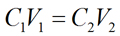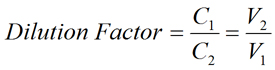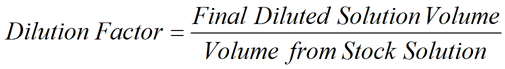PhysiologyWeb  Home  |  FAQ  |  About  |  Contact  |   - Sign InDilution Factor Calculator - Mass per Volume
Meant to be used in both the teaching and research laboratory, this calculator (see below) can be utilized to perform dilution factor calculations when working with solutions having mass per volume (i.e., mass over volume) or weight per volume (i.e., weight over volume) concentration units such as pg/mL, μg/μL, mg/mL, g/L, etc. See our Mass per Volume Solution Concentration Calculator for a definition of mass per volume or weight per volume (e.g., mg/mL, g/L, etc.). When a concentrated solution is diluted, the dilution factor may be expressed as the ratio of the concentration of stock solution to the concentration of the diluted solution. The dilution factor may also be expressed as the ratio of the volume of the final diluted solution to the initial volume removed from the stock solution. See below for the dilution factor equations.
For example, if a solution with a concentration of 1 mg/mL is diluted to yield a solution with a concentration of 1 μg/mL, the resulting dilution factor is 1000. For this particular dilution, it can also be said that the stock solution was diluted 1000-fold. As another example, if 100 mL of a stock solution is diluted with solvent/diluent to a total, final volume of 1000 mL, the resulting dilution factor is 10. It can also be said that the stock solution was diluted 10-fold. Therefore, a 10-fold dilution is the same as a dilution factor of 10. Thus, dilution by any factor X is equivalent to X-fold dilution.
Please note that the dilution factor calculator below uses the metric unit for volume (fL, pL, nL, μL, mL, and L). If you wish to convert these units to other volume units, please use our Unit Conversion Calculator.
Additional dilution factor calculators are also available and are suited to more specialized applications (see here).
If you are starting with the solid material and wish to make a solution with the concentration expressed in mass per volume or weight per volume (e.g., pg/mL, μg/μL, mg/mL, g/L, etc.), please use our Mass per Volume Solution Concentration Calculator.
Dilution factor equation - mass per volume• Dilution Factor is the factor by which the stock solution is diluted. It may be expressed as the ratio of the volume of the final diluted solution (V2) to the initial volume removed from the stock solution (V1), as shown in the equation above. Dilution factor may also be expressed as the ratio of the concentration of stock solution (C1) to the concentration of the final diluted solution (C2).
• Stock Solution Concentration (C1) is the concentration of the stock solution.
• Volume from Stock Solution (V1) is the volume to be removed (i.e., aliquoted) from the concentrated stock solution.
• Final Diluted Solution Concentration (C2) is the concentration of the final diluted solution.
• Final Solution Volume (V2) is the final volume of the diluted solution. This is the volume that results after the volume from the stock solution (V1) has been diluted with solvent or diluent to achieve a total diluted volume of the final solution (V2). Please note that the final volume refers to the total solution volume, which is the combined volume of the stock solution and the volume of solvent/diluent used for dilution.
Dilution factor calculator - mass per volume
Each calculator cell shown below corresponds to a term in the formula presented above. Two main options are available for this calculator: the dilution factor may either be calculated or specified.
Dilution factor calculated: For this calculation, enter appropriate values in all cells except the dilution factor and the one you wish to calculate. Therefore, at least three cells must have values, and the dilution factor and the parameter you wish to calculate must be blank. The value of the dilution factor and the blank cell will be calculated based on the other values entered. After a calculation is performed, the calculated cell will be highlighted and subsequent calculations will calculate the value of the highlighted cell (with no requirement to have a blank cell). However, a blank cell has priority over a highlighted cell.
Dilution factor specified: For this calculation, the following must be entered: (1) desired dilution factor; (2) either the stock concentration (C1) or final concentration (C2), but not both; and (3) either the volume from stock solution (V1) or final solution volume (V2), but not both. Therefore, two cells must be blank: (C1 or C2) and (V1 or V2). The value of the blank cells will be calculated based on the other values entered. After a calculation is performed, the two calculated cells will be highlighted and subsequent calculations will calculate the values for the highlighted cells (with no requirement to have two blank cells). However, two blank cells have priority over two highlighted cells.
For convenience, this calculator allows you to select different volume and concentration units, and the necessary conversions are carried out for you to obtain the value of the blank cell(s) in the desired unit.
 Dilution factor calculated Dilution factor specified Dilution factor Dilution factor is calculated. Enter values for any three of the four parameters shown below. The blank parameter and dilution factor will be calculated.
 Stock concentration g/mL pg ng μg mg g kg per fL pL nL μL mL L Volume from stock mL fL pL nL μL mL L Final concentration g/mL pg ng μg mg g kg per fL pL nL μL mL L Final solution volume mL fL pL nL μL mL L